# WBJEE 2015 Chemistry Paper with Solutions

WBJEE 2015 Question Paper with Solutions for Chemistry is a perfect study resource that will help candidates in understanding the different methods of solving a particular problem as well as the overall pattern of the paper. Candidates can download the WBJEE 2015 question papers and figure out the frequently occurring questions in the exam. Practising the WBJEE previous year papers will guide the students to attempt each question with the best possible approach and arrive at the solution as soon as possible to save time. Solving these papers with solutions will help the students to also understand the concepts clearly and be well prepared for the upcoming exam.

### WBJEE 2015 - Chemistry

Question 1: Match the flame colours of the alkaline earth metal salts in the Bunsen burner.

(i) Calcium (p) brick red

(ii) Strontium (q) apple green

(iii) Barium (r) crimson

1. a. a-p, b-r, c-q
2. b. a-r, b-p, c-q
3. c. a-q, b-r, c-p
4. d. a-p, b-q, c-r

Solution:

The flame colours of the alkaline earth metal salts in Bunsen burner are as shown:- Calcium gives brick red colour (a → p)

Strontium gives crimson colour (b → r)

Barium gives apple green colour (c → q)

The electron can easily be excited to higher energy levels. This results in characteristic colour during de-excitation of electrons.

Question 2: Extraction of gold (Au) involves the formation of complex ions ‘X’ and ‘Y’.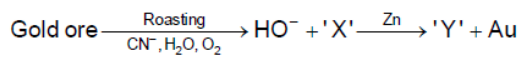‘X’ and ‘Y’ are respectively

1. a. Au (CN)2- and Zn (CN)42-
2. b. Au (CN)43- and Zn (CN)42-)
3. c. Au (CN)3- and Zn (CN)64-
4. d. Au (CN)4- and Zn (CN)3-

Solution:

Extraction of gold (Au) involves the formation of complex ions x and y. x and y are Au(CN)2- and Zn (CN)42- respectively.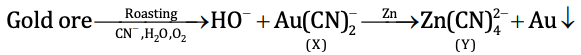Note:

Complex X is water-soluble.

More electropositive zinc displaces gold from the complex.

Question 3: The atomic number of cerium (Ce) is 58. The correct electronic configuration of Ce3+ ion is

1. a. [Xe]4f1
2. b. [Kr]4f1
3. c. [Xe]4f13
4. d. [Kr]4d1

Solution:

Z = 54 is Xe

Z = 56 is Ba

Electronic configuration of Ba = [Xe] 6s2

Z = 57 is La

Electronic configuration of La = [Xe] 5d16s2

(Electron in 5d in place of expected 4f)

But in Z = 58 (Ce) electron enters 4f and 5d' also shifts to 4f. Hence electronic configuration of Ce (58) = [Xe] 4f2 5d0 6s2

∴ Ce3+ = [Xe] 4f1

Question 4: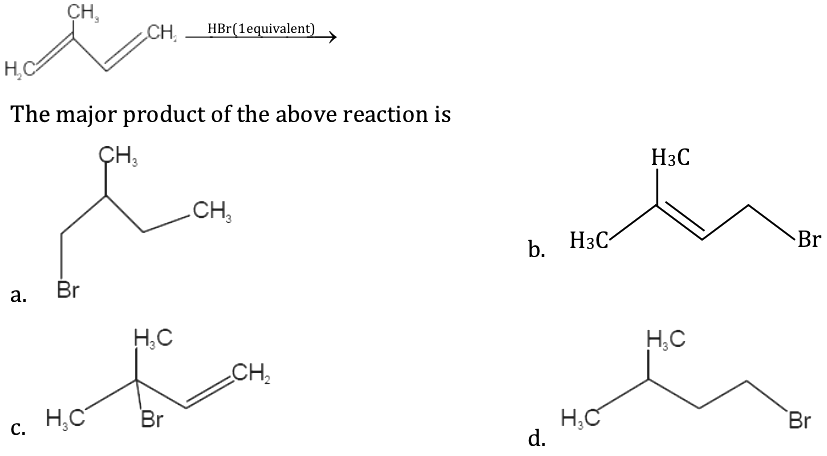Solution: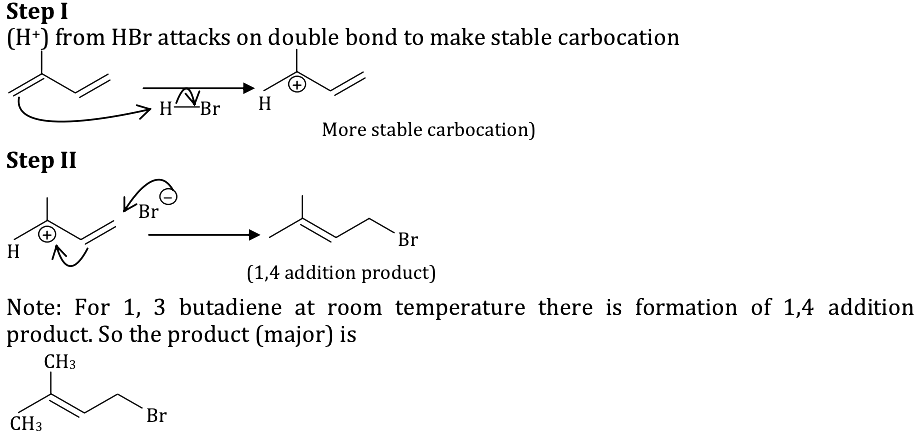Question 5: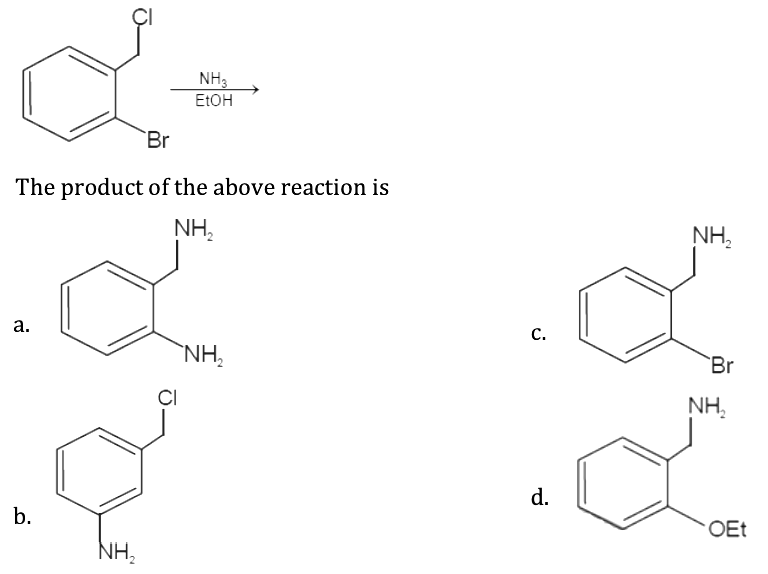Solution: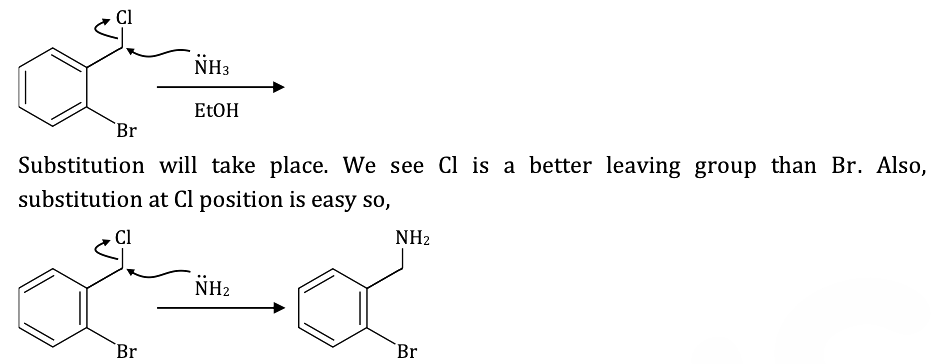Question 6: Sulphuryl chloride (SO2Cl2) reacts with white phosphorus (P4) to give

1. a. PCl5, SO2
2. b. OPCl3, SOCl2
3. c. PCl5, SO2, S2Cl2
4. d. OPCl3, SO2, S2Cl2

Solution:

Sulphuryl chloride (SO2Cl2) reacts with white phosphorous (P4) to give phosphorous pentachloride and sulphur dioxide.

10SO2Cl2 + P4 → 4PCl5 + 10SO2

Question 7: The number of lone pair of electrons on the central atoms of H2O, SnCl2, PCl3 and XeF2 respectively, are

1. a. 2,1,1,3
2. b. 2,2,1,3
3. c. 3,1,1,2
4. d. 2,1,2,3

Solution:

The number of lone pairs of electrons on central atoms of H2O, SnCl2, PCl3 and XeF2 is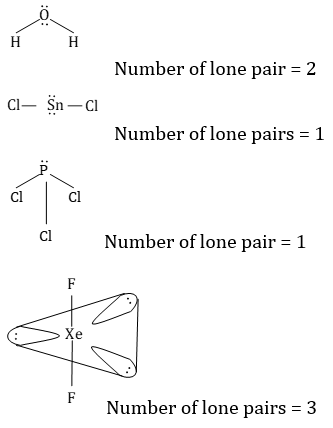Question 8: Consider the following salts: NaCl, HgCl2, Hg2Cl2, CuCl2, CuCl and AgCl. Identify the correct set of insoluble salts in water

1. a. Hg2Cl2, CuCl, AgCl
2. b. HgCl2, CuCl, AgCl
3. c. Hg2Cl2, CuCl2, AgCl
4. d. Hg2Cl2, CuCl, NaCl

Solution:

Hg2Cl2, CuCl, AgCl are insoluble in water due to high lattice enthalpy and low hydration enthalpy.

NaCl, HgCl2, CuCl2 are soluble in water due to low lattice enthalpy and high hydration enthalpy.

Question 9: In the following compound, the number of ‘sp’ hybridized carbon is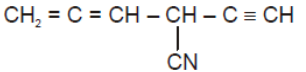1. a. 2
2. b. 3
3. c. 4
4. d. 5

Solution: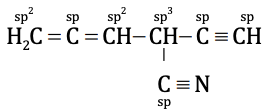Number of sp-hybridised carbon = 4

Question 10: For the reaction A + 2B → C, the reaction rate is doubled if the concentration of A is doubled. The rate is increased by four times when concentrations of both A and B are increased by four times. The order of the reaction is

1. a. 3
2. b. 0
3. c. 1
4. d. 2

Solution:

For the reaction A + 2B → C, the reaction rate is doubled, if the concentration of A is doubled. This indicates that the reaction is of first order with respect to A.

The rate is increased by four times when the concentration of both A and B is increased by four times.

This indicates that the reaction is zero-order with respect to B.

(Note – When the concentration of A is increased four times the rate is increased by four times.

Hence when the concentration of B is increased by four times, the rate is not increased.

The order of reaction = 1 + 0 = 1.

Question 11: At a certain temperature, the value of the slope of the plot of osmotic pressure (𝛑) against concentration (C in mol L–1) of a certain polymer solution is 291R. The temperature at which osmotic pressure is measured is (R is gas constant)

1. a. 271°C
2. b. 18°C
3. c. 564 K
4. d. 18 K

Solution:

The relationship between the osmotic pressure and the concentration is CRT For the plot of osmotic pressure (𝛑) against concentration (C in mol/L)

Slope RT = 291R.

T = 291 K = 18oC

Question 12: The rms velocity of CO gas molecules at 27°C is approximately 1000 m/s. For N2 molecules at 600 K the rms velocity is approximately

1. a. 2000 m/s
2. b. 1414 m/s
3. c. 1000 m/s
4. d. 1500 m/s

Solution:

Urms = √3RT / M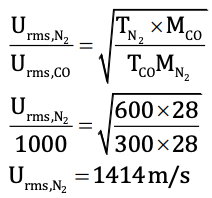Question 13: A gas can be liquefied at temperature T and pressure P provided

1. a. T = Tc and P < Pc
2. b. T < Tc and P > Pc
3. c. T > Tc and P > Pc
4. d. T > Tc and P < Pc

Solution:

When the temperature of the gas is equal to the critical temperature, the liquification is possible only when the pressure is equal to the critical pressure. Thus when the temperature is the critical temperature and the pressure is less than the critical pressure, the liquification of gas is not possible.

So, for gas to liquefy

T < Tc and P > Pc

Question 14: The dispersed phase and dispersion medium of fog respectively are

1. a. solid, liquid
2. b. liquid, liquid
3. c. liquid, gas
4. d. gas, liquid

Solution:

The dispersed phase and dispersion medium of fog respectively are liquid and gas. It is an example of liquid aerosol. Other examples of this type of aerosol include mist and clouds.

Question 15: The decreasing order of basic character of K2O, BaO, CaO and MgO is

1. a. K2O > BaO > CaO > MgO
2. b. K2O > CaO > BaO > MgO
3. c. MgO > BaO > CaO > K2O
4. d. MgO > CaO > BaO > K2O

Solution:

Alkali metal oxides are more basic than their corresponding alkaline earth metal oxides. Thus K2O is the most basic. Further, the basic character of alkaline earth metal oxides increases down the group as the electropositive character of metal increases.

BaO > CaO > MgO

Combining the two trends, the basic character increases in order

K2O > BaO > CaO > MgO

Question 16: In aqueous alkaline solution, two-electron reduction of HO2 gives

1. a. HO
2. b. H2O
3. c. O2
4. d. O2–

Solution:

In aqueous alkaline solution, two electrons reduction of HO2 gives HO

HO2 + H2O + 2e → 3OH

Question 17: Cold ferrous sulphate solution on the absorption of NO develops brown colour due to the formation of

1. a. paramagnetic [Fe(H2O)5(NO)]SO4
2. b. diamagnetic [Fe(H2O)5(N3)]SO4
3. c. paramagnetic [Fe(H2O)5(NO3)](SO4 )2
4. d. diamagnetic [Fe(H2O)4(SO4 )]NO3

Solution:

Cold ferrous sulphate solution + NO → Brown ring

For this, we need to understand the brown ring concept.

When FeSO4 reacts with H2O in presence of NO

Following reaction is obtained

FeSO4 + 5H2O + NO → [Fe(H2O)5NO] SO4

Brown ring complex

It has a magnetic moment of 3.89 BM

i.e., μ = 3.89 BM

That is 3 unpaired electrons.

Therefore formation of Brown ring complex is due to the paramagnetic nature of the complex.

Question 18: Amongst Be, B, Mg and Al the second ionization potential is maximum for

1. a. B
2. b. Be
3. c. Mg
4. d. Al

Solution:

Among B, Be, Mg and Al Boron have second-highest ionization potential. Both beryllium and magnesium belong to group II-A and boron and aluminium belongs to III A electronic configuration of Boron is 2s2 2p1

Whereas, electronic configuration of B+ is 2s2

Hence, it is difficult to remove the second electron from 2s2 shell as half-filled and fully filled orbitals are more stable than others.

Question 19: In a mixture, two enantiomers are found to be present in 85% and 15% respectively. The enantiomeric excess (e, e)

1. a. 85%
2. b. 15%
3. c. 70%
4. d. 60%

Solution:

Two enantiomers are found to be present in 85% and 15% respectively.

Now, 15% will form a racemic mixture with the other 15%.

Now enantiomeric excess = (85 - 15)% = 70%

Question 20: 1,4-dimethyl benzene on heating with anhydrous AlCl3 and HCl produces

1. a. 1,2-dimethylbenzene
2. b. 1,3-dimethyl benzene
3. c. 1,2,3-trimethylbenzene
4. d. Ethylbenzene

Solution:

1,4-dimethyl benzene ring on heating with anhydrous AlCl3 and HCl produces 1,3- dimethyl benzene.

The net reaction is the isomerisation of di-substituted benzene.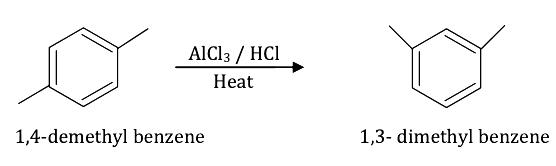Question 21: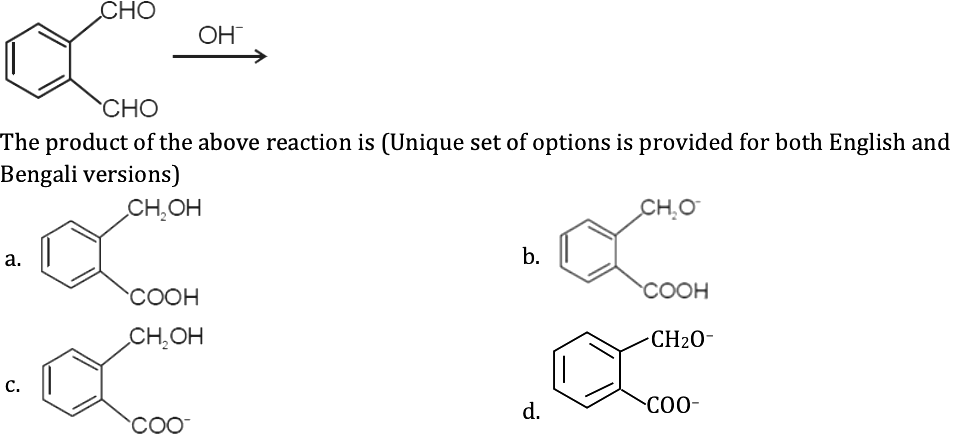Solution: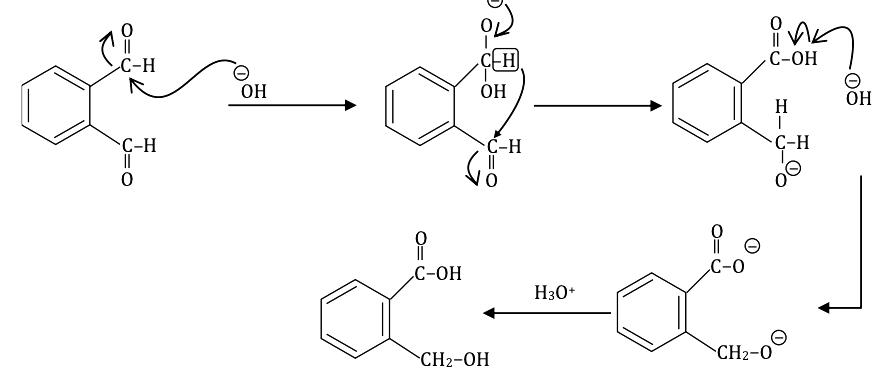Here intermolecular Cannizzaro reaction takes place.

Question 22: Suppose the mass of a single Ag atom is ‘m’. Ag metal crystallizes in fcc lattice with a unit cell of length ‘a’. The density of Ag metal in terms of ‘a’ and ‘m’ is

1. a. 4m / a3
2. b. 2m / a3
3. c. m / a3
4. d. m / 4a3

Solution:

The edge length of the unit cell = a

The volume of unit cell = a3

One FCC unit cell contains 4 Ag atoms as [as 6 atoms present at face centre and 8 at corner]

Contribution at face = 1 / 2

Contribution at corner = 1 / 8

Rank (z) = (1 / 2) * 6 + (1 / 8) * 8 = 3 + 1 = 4

Mass of one Ag atom = m.

Mass of 4 Ag atoms = 4 m.

Density = mass / volume

d = 4m / a3

Question 23: For the reaction 2SO2(g) + O2(g) ⇌ 2SO3(g) at 300K, the value of ΔG0 is – 690.9R. The equilibrium constant value for the reaction at that temperature is (R is gas constant)

1. a. 10 atm–1
2. b. 10 atm
3. c. 10
4. d. 1

Solution:

Given reaction

2SO2(g) + O2(g) ⇌ 2SO3(g)

ΔG0 = – 690.9R --- (1)

at T = 300 K

Gibbs free energy in terms of the equilibrium constant is given as

ΔG0 = -RT ln keq.

Using equation (1)

– 690.9R = – RT ln Keq.

– 690.9 R = – R (300) ln Keq.

ln Keq. = 690.9 / 300 = 2.303

Using ln keq. = 2.303 log10 Keq.

2.303 log10 Keq. = 2.303

log10 Keq. = 1

K = 101 = 10

Unit of K

K = (atm)Δn ......(3)

2SO2(g) + O2(g) ⇌ 2SO3(g)

Δn = 2 – 3 = –1 ...... (2)

Using (2) in (3)

K = (atm)–1

Therefore K = 10 atm–1

Question 24: At a particular temperature the ratio of equivalent conductance to specific conductance of a 0.01 (N) NaCl solution is

1. a. 105 cm3
2. b. 103 cm3
3. c. 10 cm3
4. d. 105 cm2

Solution:

The relation between equivalent conductance and specific conductance is given as

λ = [K * 1000] / C

Here C = 0.01 N (given)

(λ / K) = 1000 / C = 1000 / 0.01 = 105 cm3eq-1

λ = [Ω-1cm2eq-1] / [Ω-1cm-1] = cm3eq-1

Question 25: The units of surface tension and viscosity of liquids are respectively

1. a. kg m–1s–1, Nm–1
2. b. kg s–2, kg m–1 s–1
3. c. Nm–1, kg m–1s–2
4. d. kg s–1, kg m–2 s–1

Solution:

Surface tension (γ) is given as work done by Area.

r = Δω / ΔA = J / m2 = Kgm2s-2 / m2 = Kgs-2

Work done in Joules

The area in M2

Also 1J = 1 Kgm2s-2

Viscous drag (F) given as

F ∝ A

F ∝ dv / dx

F = 𝜂A (dv / dx)

(Viscosity)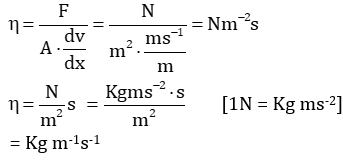Question 26: The ratio of volumes of CH3COOH 0.1 (N) to CH3COONa 0.1 (N) required to prepare a buffer solution of pH 5.74 is (given: pKa of CH3COOH is 4.74)

1. a. 10 : 1
2. b. 5 : 1
3. c. 1 : 5
4. d. 1 : 10

Solution:

pH = 5.74

pKa = 4.74

Let volume of acid solution = XL

Volume of salt solution = YL

pH = buffer is given as

pH = pKa + log [[Salt] / [Acid]]

CH3COOH + NaOH → CH3COONa + H2O

log10 {[CH3COONa] / [CH3COOH]} = 5.74 - 4.74

{[CH3COONa] / [CH3COOH]} = 1 / 10 ---- (1)

Using Molarity, (M) = Moles (n) / Volume v

Moles (n) = Mv

Total volume = x + y

Number of moles of CH3COONa = 0.1 × x

Number of moles of CH3COOH = 0.1 y

Putting these values in (1),

[0.1x / x + y] / [0.1y / x + y] = 1 / 10

(x / y) = 1 / 10

Question 27: The reaction of methyltrichloroacetate (Cl3CCO2Me) with sodium methoxide (NaOMe) generates

1. a. Carbocation
2. b. Carbene
3. c. Carbanion
4. d. Carbon radical

Solution:

The reaction of methyl trichloroacetate (Cl3CCO2Me) with sodium methoxide (NaOMe) generates carbene. A carbene is neutral species having a carbon atom with two bonds and two electrons.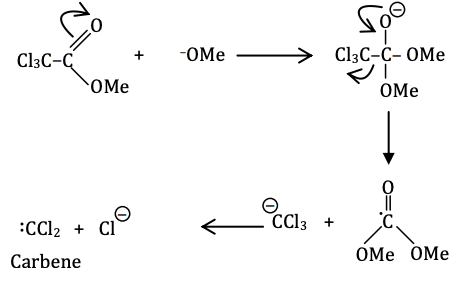Question 28: Best reagent for nuclear iodination of aromatic compounds is

1. a. KI/CH3COCH3
2. b. I2/CH3CN
3. c. KI/CH3COOH
4. d. I2/HNO3

Solution:

Best reagent for nuclear iodination of aromatic compounds is I2/HNO3. During iodination of aromatic compounds, HI is produced which is a strong reducing agent and can reduce iodobenzene back to benzene. To prevent this, oxidising agent such as iodic acid, nitric acid or mercuric oxide is used.

Question 29: In the Lassaigne’s test for the detection of nitrogen in an organic compound, the appearance of a blue coloured compound is due to

1. a. ferric ferricyanide
2. b. ferrous ferricyanide
3. c. ferric ferrocyanide
4. d. ferrous ferrocyanide

Solution:

Carbon and nitrogen present in the organic compound on fusion with sodium metal give sodium cyanide (NaCN) soluble in water.

This is converted into sodium ferrocyanide by the addition of a sufficient quantity of ferrous sulphate.

Ferric ions generated during the process react with ferrocyanide to form Prussian blue precipitate of ferric ferrocyanide.

Na + C + N → NaCN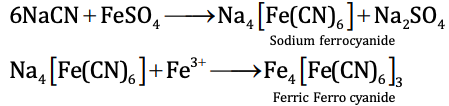Question 30: In the following reaction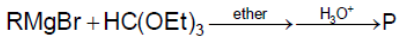The product ‘P’ is

1. a. RCHO
2. b. R2CHOEt
3. c. R3CH
4. d. RCH(OEt)2

Solution:

In the reaction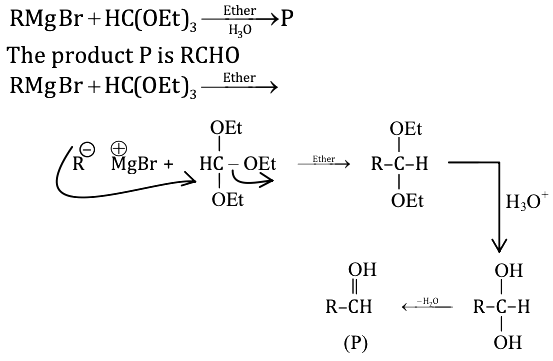Question 31: Addition of sodium thiosulphate solution to a solution of silver nitrate gives ‘X’ as a white precipitate, insoluble in water but soluble in excess thiosulphate solution to give ‘Y’. On boiling in water, ‘Y’ gives ‘Z’. ‘X’, ‘Y’ and ‘Z’ respectively, are

1. a. Ag2S2O3, Na3[Ag(S2O3)2], Ag2S
2. b. Ag2SO4, Na[Ag(S2O3)2], Ag2S2
3. c. Ag2S2O3, Na5[Ag(S2O3)3], AgS
4. d. Ag2SO3, Na3[Ag(S2O3)2], Ag2O

Solution:

The addition of sodium thiosulphate solution to a solution of silver nitrate gives 'X' as a white precipitate, insoluble in water but soluble in excess thiosulphate solution to give 'y'

On boiling in water y gives z x, y and z respectively are: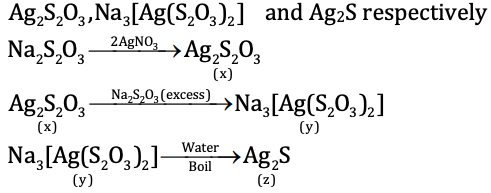Question 32: At temperature of 298 K the emf of the following electrochemical cell Ag(s) | Ag+ (0.1 M) | |Zn2+(0.1M) | Zn(s) will be (given Eocell = – 1.562 V)

1. a. – 1.532 V
2. b. – 1.503 V
3. c. 1.532 V
4. d. – 3.06 V

Solution:

From the given cell, the cell reaction is

2Ag(s) + Zn2+ (0.1M) → 2Ag+ (0.1) + Zn(s)

The Nernst equation is

Ecell = E0cell - (0.0591 / n) log {[Ag+]2 / [Zn2+]}

= (-1.562) - (0.0591 / 2) log {[0.12 / 0.1]}

= (-1.562) - (0.0591 / 2) log 10-1

= (-1.562) + (0.0591 / 2)

= - 1.562 + 0.02955

= –1.532 V

Question 33: For the reaction X2Y4(I) → 2 XY2(g) at 300 K the values of ΔU and ΔS are 2 kCal and 20 Cal K–1 respectively. The value of ΔG for the reaction is

1. a. – 3400 Cal
2. b. 3400 Cal
3. c. – 2800 Cal
4. d. 2000 Cal

Solution:

Reaction

X2Y4(I) → 2 XY2(g)

Δn = ng(P) - ng(R)

= 2 – 0

= 2

We know ΔH = ΔU + Δng RT

= 2 + [2 * 2 * 300] / 1000 [R = 2cal K-1 mol-1 and T = 300K]

= 3.2Kcal

We know

ΔG = ΔH - TΔS

= 3.2 * 10-3 - 300 * 20 [ΔS = 20calK-1]

= 3.2 * 10-3 - 6 * 103

= – 2800 Cal

Question 34: The total number of aromatic species generated in the following reactions is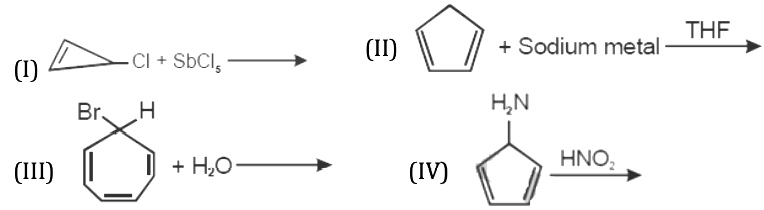1. a. Zero
2. b. 2
3. c. 3
4. d. 4

Solution:

For a species to be aromatic

(1) It should be planar

(2) Conjugation present

(3) Should have (4n + 2) 𝛑 electrons.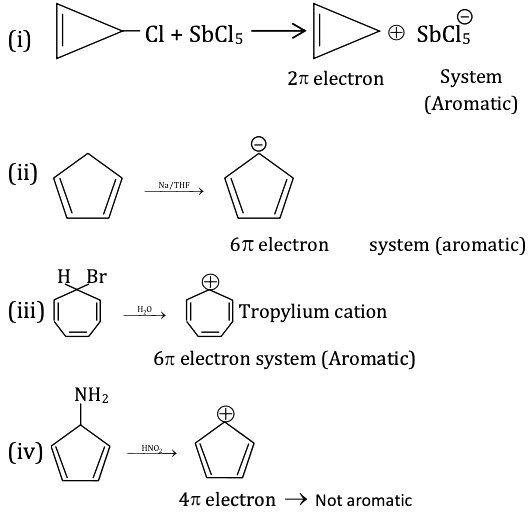Question 35: Roasted copper pyrite on smelting with sand produces

1. a. FeSiO3 as fusible slag and Cu2S matte’
2. b. CaSiO3 as infusible slag and Cu2O matte’
3. c. Ca3(PO4)2 as fusible slag and Cu2S matte’
4. d. Fe3(PO4)2 as infusible slag and Cu2S matte’

Solution:

Roasted copper pyrite on smelting with sand produces FeSiO3 as fusible slag and Cu2S as matte

2CuFeS2 + O2 → Cu2 S + 2FeS + SO2

2FeS + 3O2 → 2 FeO + SO2

FeO + SiO2 → FeSiO3 → FeSiO3 (slag)

FeSiO3 is fusible slag Cu2S as matte.

Question 36: Ionization potential values of noble gases decrease down the group with an increase in atomic size. Xenon forms binary fluorides by the direct reaction of elements. Identify the correct statement(s) from below

1. a. Only the heavier noble gases form such compounds
2. b. It happens because noble gases have higher ionization energies.
3. c. It happens because the compounds are formed with electronegative ligands.
4. d. Octet of electrons provides stable arrangements.

Solution:

Option a and c are correct

(a) Only the heavier noble gases form such compounds as they have relatively lower values of ionisation enthalpies.

(c) It happens because the compounds are formed with electronegative ligands. Option b and d are incorrect.

(b) Since the noble gases have higher ionisation energies the tendency for compound formation will be lower.

(d) Octet of electrons provides stable arrangements. During compound formation, the octet is broken and stability is lost.

Question 37: Optical isomerism is exhibited by (ox = oxalate anion; en = ethylenediamine)

1. a. cis-[CrCl2(ox)2]3–
2. b. [Co(en)3]3+
3. c. trans-[CrCl2(ox)2]3–
4. d. [Co(ox)(en)2]+

Solution:

1. Answer: (a, b, d)

(a) cis-[CrCl2(ox)2]3+

(b) [Co(en)3]3+

(c) [Co(ox)(en)2]+ trans-[CrCl2(ox)2]3– isomer optically inactive (super imposable mirror images and plane of symmetry)

cis-[CrCl2(ox)2]3–, [Co(en)3]3+ and [Co(ox)(en)2]+ exhibited optical isomerism.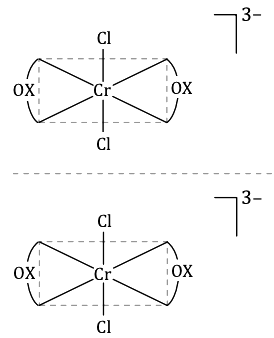Question 38: The increase in the rate constant of a chemical reaction with increasing temperature is (are) due to the fact(s) that

1. a. The number of collisions among the reactant molecules increases with increasing temperature.
2. b. The activation energy of the reaction decreases with increasing temperature.
3. c. The concentration of the reactant molecules increases with increasing temperature.
4. d. The number of reactant molecules acquiring the activation energy increases with increasing temperature.

Solution:

1. Answer: (a, d)

The increase in the rate constant of a chemical reaction with increasing temperature is (a) The number of collisions among the reactant molecules increases with increasing temperature.

(d) The number of reactant molecules acquiring the activation energy increases with increasing temperature.

Question 39: Within the list shown below, the correct pair of structures of alanine in pH ranges 2-4 and 9-11 is

(I) H3N+ – CH(CH3)CO2H

(II) H2N – CH(CH3)CO2

(III) H3N+ – CH(CH3)CO2

(IV) H2N+ – CH(CH3)CO2H

1. a. I, II
2. b. I, III
3. c. II, III
4. d. III, IV

Solution:

As the isoelectric point of Alanine is 6.1

At pH below pI it has NH3

At pH above pI it has COO-

for pH → 2 – 4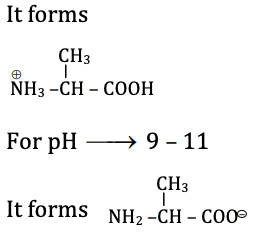Question 40: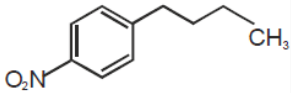Identify the correct method for the synthesis of the compound shown above from the following alternatives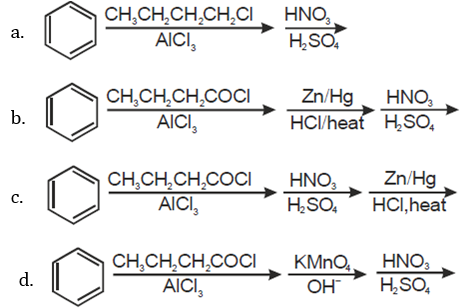Solution:

The synthesis is as shown

Friedel crafts acylation of benzene with butanol chloride in presence of anhydrous aluminium chloride gives 1-phenylbutan-1-one

Clemmensen’s Reduction with Zn/Hg in presence of HCl gives butyl benzene. Nitration with nitrating mixture gives 1-butyl-4-nitrobenzene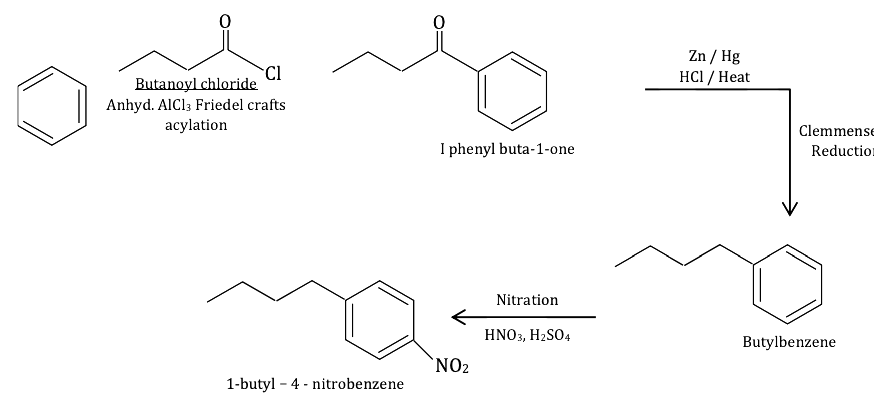### WBJEE 2015 Chemistry Question Paper with Solutions